### Test: PSAT Math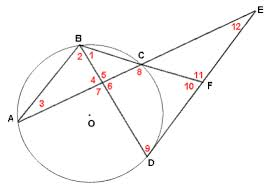1 In the figure above that includes Circle O, the measure of angle BAC is equal to 35 degrees, the measure of angle FBD is equal to 40 degrees, and the measure of arc AD is twice the measure of arc AB. Which of the following is the measure of angle CEF? The figure is not necessarily drawn to scale, and the red numbers are used to mark the angles, not represent angle measures.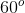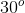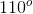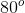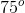1/13 questions

0%

## Access results and powerful study features!

Take 15 seconds to create an account.